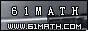Today is . WELCOME: huohai3983/44663  Home | Reg Login | All user | setHomePage | BookmarkHome | Math Test Online | Addition | Subtraction | Multiplication | Division | Time | Worksheet(JPG/PDF) | CAI | Flash Games |Recommendation articleArticle searchHome--maths--Second Grade--Subtracting Hundreds

Subtracting Hundreds

Subtracting Hundreds

Subtracting hundreds such as (800 - 200 = 600) is like subtracting quantities of other items. In this case 2 hundreds are subtracted from 8 hundreds to leave 6 hundreds. This would be similar to 2 oranges being subtracted from 8 oranges to leave 6 oranges.

Each digit of a number in our number system has a different place value (ones, tens, hundreds, etc.). Always subtract within the same place value column when subtracting numbers.

#### Find the Difference between the two Numbers.

 =

2006-12-14 22:45:05
Viewed 2874 times CloseGreatmathsites

### Translate

 Thank you to online math channels' Mathematics Department for hosting these lessons. Copyright © 2006 - 2008 GZtrain Inc. All Rights Reserved